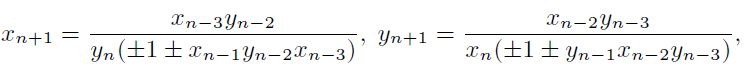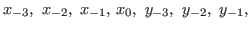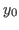## Elsayed M. Elsayed : On the solutions and periodicity of some rational systems of difference equations p. 159-171

### Abstract:

In this paper we deal with the form of the solutions and the periodicity nature of the following systems of nonlinear difference equationswhere the initial conditionsandare nonzero real numbers.

Key Words: difference equations, periodic solutions, system of difference equations.

2010 Mathematics Subject Classification: 39A10.